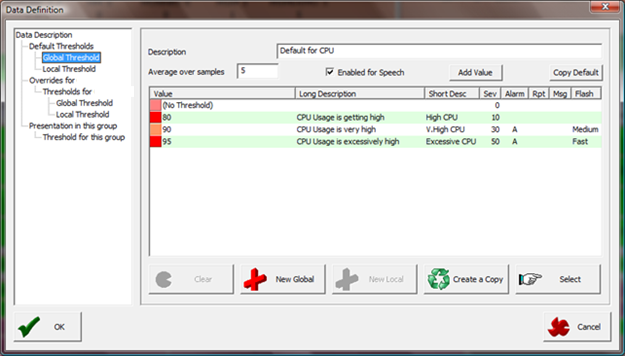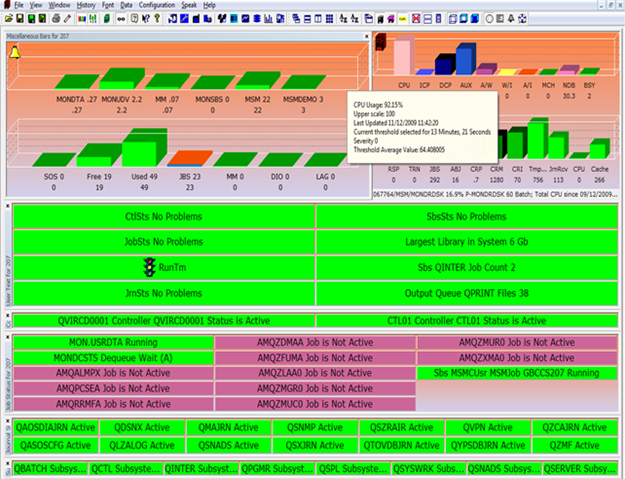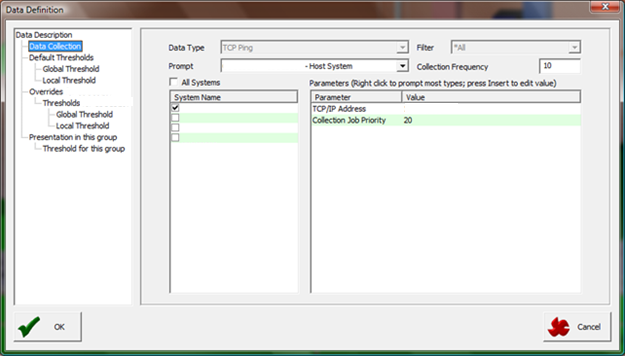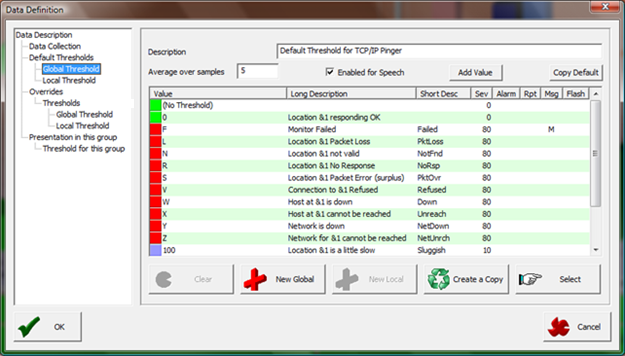Thresholds have a mechanism to average over a number of samples. Therefore, when a bar has a threshold attached, it can show you the average over a number of samples. The value displayed is the current value. Hovering over the bar shows the threshold average value across the samples.

## Example 1 - CPU

CPU has a threshold averaged over 5 samples, with an alert value of ten.

If the first 3 samples are 5, 5, and 5, no threshold would be triggered as 5 + 5 + 5 ÷ 3 = 5.

If the next value is 50, the threshold will be triggered, since 5 + 5 + 50 ÷ 3 = 20. If the next value had been 17 instead of 50, the threshold wouldn't have been triggered because 5 + 5 + 17 ÷ 3 = 9.

Following is an example based on CPU, set to be averaged across 5 samples. Note the setting on the Global Threshold (Average over samples) window:You can see below, that even though CPU Usage is currently 92% the threshold has not been triggered because the average value is only 64%, which is below the first trigger value of 80%:So, using Average over samples is a handy way of removing alerts caused by one-off peaks.

## Example 2 – TCP Ping

Following is an example based on a TCP Ping, created to be averaged across 5 samples. This definition also has a Collection Frequency parameter, which allows greater flexibility.

In this example, 10 collections = 300 seconds and 5 samples = 1500 seconds, so the average is covering a period of 25 minutes.

Note the setting on the Data Collection (Collection Frequency) window:Note the setting on the Global Threshold (Average over samples) window:You can see below, that in this case the threshold has been triggered because the average value is 209, which is greater than second trigger value of 200:Still have questions? We can help. Submit a case to Technical Support.